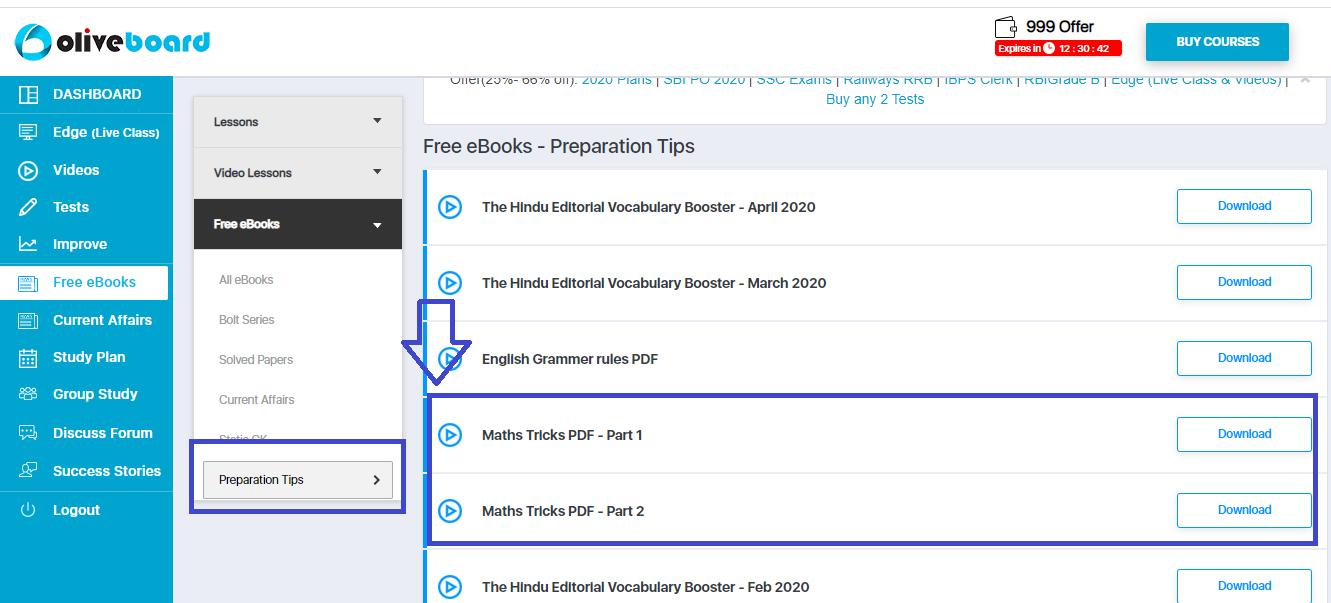# Quicker Maths Tricks PDF [Topic-Wise] – Learn Fast Calculation Tricks

Quantitative Aptitude or Numerical Ability section does not need any introduction. You know it forms a significant part of almost every Bank/Insurance/SSC/Railways/Government exam. Lakhs and Lakhs of candidates appear in this exams every year. To get your desired job in this pool of competition, your approach should be unique and different.To help you with that, Here we bring you Short Maths Tricks PDF available for free download, which will make your preparation comprehensive and calculations faster. Short tricks reduce the time taken to solve a question to almost a quarter of the initial time. Let’s start and see how these tricks are helpful.

### Part – 1### Part – 2Step 2: Register/Login on to the Free E-Books Page of Oliveboard (It is 100% free, You just enter your valid email id and a password to be able to download the Maths tricks PDF).

Step 3: After Logging in, you will be able to see the eBook Maths Tricks PDf (part 1 & 2). Click on the download button and you will be able to access the PDF.## Maths Tricks PDF – Solved Examples

Here are a few direct tricks and their applications.

### SHORTCUT TRICK -1 (Distance Calculation Between 2 points)

To calculate the distance between 2 points when an object is traveling to and fro from one point to another, and just the speed and time taken is given, you can use the below formula directly to calculate the distance.

d= (t1+t2)((a+b)/ab)

Q1) If a man takes 3 hrs and 5 hrs respectively in going from point A to B then from B to A at 6km/hr and 8 km/hr respectively. What will be the distance between points A & B?

Ans: Directly apply the formula to get the answer: d= (t1+t2)((a+b)/ab)

d= (3+5)((6+8)/48) = 8*(14/48) = 14/6 km.

Where T1 & T2 are the time taken, a & b are the respective speeds and d = distance.

### SHORTCUT TRICK -2 (Multiplication By 11)

If a number is given to be multiplied by 11, you can use the following method to calculate the answer. Suppose you have 79*11. Put the number of 79 at units and 100’s place and put the total of that number in between. if the total exceeds 10 add 1 the digit at 100’s place thus bringing your answer.

ab*11 = a(a+b)b,

If a+b>10 add 1 to ‘a’ and keep the rest term intact. below is the sample question.

Q2) Calculate what is 79*11.

Ans: If you go by the regular formula you might not be able to do it verbally. This trick is applicable only when a number is said to be multiplied by ’11’ Here is a direct trick to solve this multiplication.

79*11 = 7() 9 = 7(16)9 = 869

### SHORTCUT TRICK – 3 (Calculation of Multiple Interest on CI)

If a loan is taken for more than 1 year and the rate of interest in varying for different years, you can directly use the formula given here to calculate the answer.

Amount = P[1+R1/100] [1+R2/100] [1+R3/100] where R1 = rate of interest for 1st year, R2 for the second year and so on.

Q3) If 40000 is given as loan for a period of 3 years with the interest rates 6%, 8% and 9% for the 1st, 2nd and 3rd year respectively. What is the total amount that has to be paid at the end of 3 years?

Ans: By going with the regular method you will have to calculate the CI for each year and then add the CI of next year to the previous years’ number.

Here is a direct trick to avoid this:

Amount = P[1+6/100] [1+8/100] [1+9/100] where R1 = rate of interest for 1st year(6%), R2 (8%) for the second year and so on. Put the values and get the answer. Repeat it for any other similar case.

To get a complete list of such short tricks and formulas,  download the free eBook now.